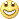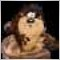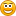# Stochastic Momentum Indicator (Index) - page 2204

Hi Kalenzo (Mr Holmes!)What is the difference between T3 TRIX in this forum and Metastock TRIX?

see these

Files:
ms.jpg 41 kb
mt.gif 10 kb1411

Hi Kalenzo (Mr Holmes!)What is the difference between T3 TRIX in this forum and Metastock TRIX?

see these

Our is betterlol just kiddingPost the metastock formula, i will check it.204

I can't get formula.

I think metastock gives some formula no all.113

If anybody interested in trying that method taken from Moneytec

I trade 18 pairs, use 4h charts and only spend 1h per day reviewing trades. So far have only used 0.5 lots with maximum stops 150 pt (2 - 5% risk). Strike rate is 77%. If I can make over \$7500 per month trading half lots risking less than 5%, its easy to see possibility of making \$20k or more per month soon. I'm increasing now to full lots, will move on from there.

I know.... early days, will need to see if I can continue to exceed 1500 pt per month.

No-one will believe 238% profit in 4 months. Take 4h GBPJPY chart and add 25 period Hull Moving Average (HMA) with slope coloured for up and down. Add Stochastic Momentum Indicator (SMI) with settings 39,6,75 and signal line 15. See if the chart tells you anything!.75

licho:
If anybody interested in trying that method taken from Moneytec

SMI does not make any sence, may be setting are not correct. HMA is good but it needs some validation or filter indicator.

Thanks90

I have the tradestation code for TRIX...and IMHO it is a good indicator. the code is divided into a function and an indicator. hope somebody will be able to convert it.

Function::::::::::::

Price( numericseries ),

Length( numericsimple ) ; { this input assumed to be a constant >= 1 }

variables:

TRIXRaw( 0 ) ;

TRIXRaw = XAverage( XAverage( XAverage( Log( Price ), Length ) , Length ) , Length ) ;

if CurrentBar > 1 then

TRIX = ( TRIXRaw - TRIXRaw ) * 10000 ;

Indicator::::::::::::::::

inputs:

Price( Close ),

Length( 9 ),

ColorNormLength( 14 ), { Number of bars over which to normalize the indicator

UpColor( Yellow ), { Color to use for indicator values that are relatively high

over ColorNormLength bars. }

DnColor( Red ), { Color to use for indicator values that are relatively low

over ColorNormLength bars. }

GridForegroundColor( Black ) ; { Color to use for numbers in RadarScreen cells

when gradient coloring is enabled, that is, when both UpColor and DnColor are

set to non-negative values. }

{ Set either UpColor and/or DnColor to -1 to disable gradient plot coloring.

When disabled, Plot1 color is determined by settings in indicator properties

dialog box. Plot2 (ZeroLine) color always comes from indicator properties

dialog box. }

variables:

ApplicationType( 0 ),

TRIXValue( 0 ),

ColorLevel( 0 ) ;

if CurrentBar = 1 then

ApplicationType = GetAppInfo( aiApplicationType ) ;

TRIXValue = TRIX( Price, Length ) ;

Plot1( TRIXValue, "TRIX" ) ;

Plot2( 0, "ZeroLine" ) ;

if UpColor >= 0 and DnColor >= 0 then

begin

ColorLevel = NormGradientColor( TRIXValue, true, ColorNormLength, UpColor, DnColor ) ;

if ApplicationType = 1 then { study is applied to a chart }

SetPlotColor( 1, ColorLevel )

else if ApplicationType > 1 then { study is applied to grid app }

begin

SetPlotColor( 1, GridForegroundColor ) ;

SetPlotBGColor( 1, ColorLevel ) ;

end ;

end ;

if TRIXValue crosses over 0 then

else if TRIXValue crosses under 0 then

Alert( "Indicator turning negative" ) ;

----------------------------------------------------------------

The XAverage function is a weighted moving average of the prices of the last length bars. This function returns the current value of the exponentially smoothed moving average.90

Another TRIX formula from Tradestation. made by some programmer. has TRIX and a signal line with it.

inputs:

TRIXLength( 5 ),

SigLineXMALen( 3 ) ;

variables:

EMA1( 0 ),

EMA2( 0 ),

EMA3( 0 ),

TRIXLine( 0 ),

SignalLine( 0 ) ;

EMA1 = XAverage( Close, TRIXLength ) ;

EMA2 = XAverage( EMA1, TRIXLength ) ;

EMA3 = XAverage( EMA2, TRIXLength ) ;

if EMA3 0 then

{ Ensure not about to divide by zero }

TRIXLine = 10 * ( EMA3 - EMA3 ) / EMA3 ;

SignalLine = XAverage( TRIXLine, SigLineXMALen ) ;

Plot1( TRIXLine, "TRIXLine" ) ;

Plot2( SignalLine, "SignalLine" )1411

licho:
If anybody interested in trying that method taken from Moneytec

licho mabye u will tell us what do u see when u plot those 2 on chart?90

is anyone working on this one??

gabroo_munda:
Another TRIX formula from Tradestation. made by some programmer. has TRIX and a signal line with it.

inputs:

TRIXLength( 5 ),

SigLineXMALen( 3 ) ;

variables:

EMA1( 0 ),

EMA2( 0 ),

EMA3( 0 ),

TRIXLine( 0 ),

SignalLine( 0 ) ;

EMA1 = XAverage( Close, TRIXLength ) ;

EMA2 = XAverage( EMA1, TRIXLength ) ;

EMA3 = XAverage( EMA2, TRIXLength ) ;

if EMA3 0 then

{ Ensure not about to divide by zero }

TRIXLine = 10 * ( EMA3 - EMA3 ) / EMA3 ;

SignalLine = XAverage( TRIXLine, SigLineXMALen ) ;

Plot1( TRIXLine, "TRIXLine" ) ;

Plot2( SignalLine, "SignalLine" )113

I have tried to work sth out but to be honest I do not see anything more valuable then using other indicator combinations. Probably you could use each indicator successfuly once you master it.

Once again it has only ensure me that my turn to S/R analysis is the right one